### Day 10 - Quiz 2 - 08.29.14

 Bell Ringer Ask any questions you may have about the quiz! Review Finding limits using a table Finding limits using a graph Epsilon-Delta Limit Proofs Limit Properties Dividing Out/Rationalizing Techniques Functions that agree at all but one point One-sided Limits Infinite Limits Lesson Quiz 2! Find the values of the constants a and b such that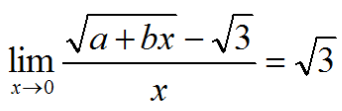Consider the function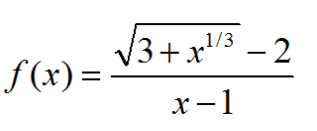Find the domain of f. Calculate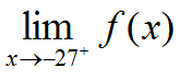Calculate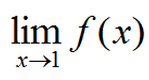Determine all value of the constant a such that the following function is continuous for all real numbers.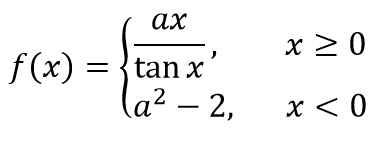Exit Ticket The function f and its graph are shown below: Calculate the limit of f(x) as x gets closer to 2 from the left side. Which value is greater? the limit of f(x) as x goes to 1 f(1) Explain your answer. At what value(s) of c on the interval [0, 4] does the limit of f(x) as x goes to c not exist? Explain your answer.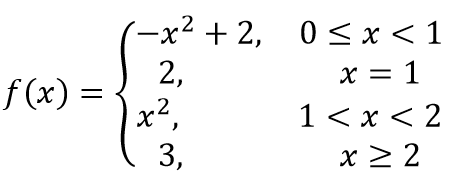Lesson Objective(s) How are the concepts in chapter 1 related? Standard(s) APC.2 Define and apply the properties of limits of functions. Limits will be evaluated graphically and algebraically. Includes: ​limits of a constant ​limits of a sum, product, and quotient ​one-sided limits ​limits at infinity, infinite limits, and non-existent limits*APC.3Use limits to define continuity and determine where a function is continuous or discontinuous.Includes:​continuity in terms of limitscontinuity at a point and over a closed interval​application of the Intermediate Value Theorem and the Extreme Value Theorem​geometric understanding and interpretation of continuity and discontinuityAPC.4Investigate asymptotic and unbounded behavior in functions.Includes:describing and understanding asymptotes in terms of graphical behavior and limits involving infinity